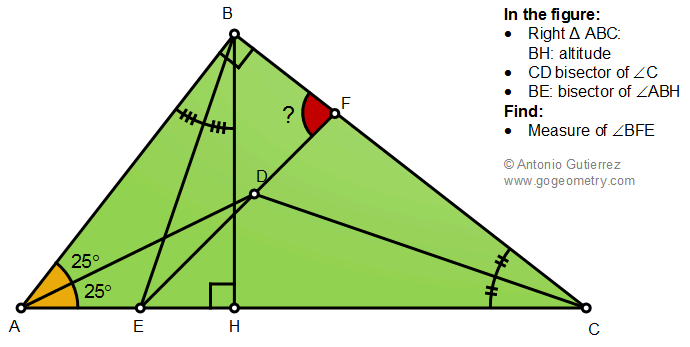# Geometry Problem 1488: Right Triangle, Altitude, Angle Bisectors, Measurement

The figure shows a right triangle ABC with altitude BH. CD and BE are the bisectors of angles C and ABH, respectively. If angle BAD = angle DAC = 25 degree, find the measure of the angle BFE.